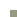## [Tweets]

15/11/2019 6:01am
RT @AandKrobotics: Beyond buzzwords. Our Senior Developer, @l_pitonakova will be talking robots, #AI and #machinelearning and real-world ap…
26/09/2019 7:14am
Day one at #ScirocChallenge: social #robots and humans meet in a shopping mall [LINK]

# [Basic Matrix Operations in MATLAB]Date: Nov 2011

Here I provide a quick look up of commonly used matrix functions in MATLAB. All of these are compatible with Octave as well.

### Creating matrices

``` % Produce 2x3 matrix of ones A = ones(2,3) % Produce 2x3 matrix of twos A = 2*ones(2,3) % Produce 2x3 matrix of 0s A = zeros(2,3) % Produce 2x3 matrix of random numbers A = rand(2,3) % Produce 2x3 matrix of gaussian numbers with mean 0 and variation 1 A = randn(2,3) % Produce large vector of gaussian numbers A = -6 + sqrt(10)*(randn(1,10000)); % Print histogram hist(A) % Produce 3x3 matrix of random numbers from <-1;1> A = rand(3,4); % 5x5 identity matrix I = eye(5) % Magic square (all columns, rows, diagonals sum up to same number) MAGIC = magic(3) ```

### Operations on matrices

``` % Everything in 2nd column A (:,2) % Everyting from 1st and 3rd row, all columns A([1 3],:) % Append another column vector A = [A, [100; 101; 102; 103]] % All elements of A into single column vector B = A(:) % Invert matrix C = pinv(A) % Transpose matrix D = C' % Element-wise multiplication (works with other signs too): E = rand(2,3) F = 2*ones(2,3) G = E .* F % ElementwiseLogarithm of matrix log(E) % Elementwise Exponentiaion exp(E) % Element wise minus E = -E % Elementwise Absolute values abs(E) % Elementwise adding e = [2;4;5;7] H = e + ones(length(e),1) I = E + 1 % Max value of vector a = [1 34 6 2] val = max(a) [val, index] = max(a) % Element-wise comparison (returns true/false) a < 3 % Element-wise comparison (returnse elements) find(a < 3) % Add up elements summation = sum(a) % Mulitply elements product = prod(a) % Floor elements FLOORING = floor(a) % Ceil elements CEILING = ceil(a) % Column- and row- wise maximums where last argument is dimension columnWiseMaximums = max(A,[],1) rowWiseMaximums = max(A,[],2) maximumElement = max(A(:)) % SumUp elements to create vector sumOfColumns = sum(MAGIC,1) sumOfRows = sum(MAGIC,2) % Diagonal sum, first multiply by identity matrix to make all % elements expect of diagonal 0 MAGIC .* eye(3) sumOfDiagonal = sum(sum(MAGIC.*eye(3))) ```

### Measuring matrices

``` % Size of matrix S = size(A) % Size of rows S = size(A,1) % Size of columns S = size(A,2) % Size of longer dimension S = length(A) ```

#### pyCreeper

The main purpose of pyCreeper is to wrap tens of lines of python code, required to produce graphs that look good for a publication, into functions. It takes away your need to understand various quirks of matplotlib and gives you back ready-to-use and well-documented code.

#### Novelty detection with robots using the Grow-When-Required Neural Network

The Grow-When-Required Neural Network implementation in simulated robot experiments using the ARGoS robot simulator.

#### Fast Data Analysis Using C++ and Python

C++ code that processes data and makes it available to Python, significantly improving the execution speed.

#### Designing Robot Swarms

This project looks at the challenges involved in modeling, understanding and designing of multi-robot systems.

#### Robustness in Foraging E-puck Swarms Through Recruitment

Swarms of five e-puck robots are used in a semi-virtual environment, facilitated by the VICON positioning system. Recruitment can make swarms more robust to noise in robot global positioning data.